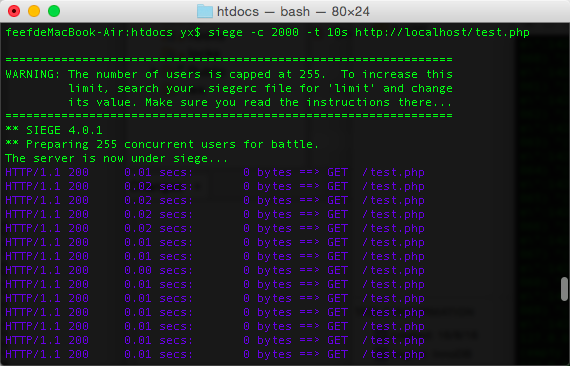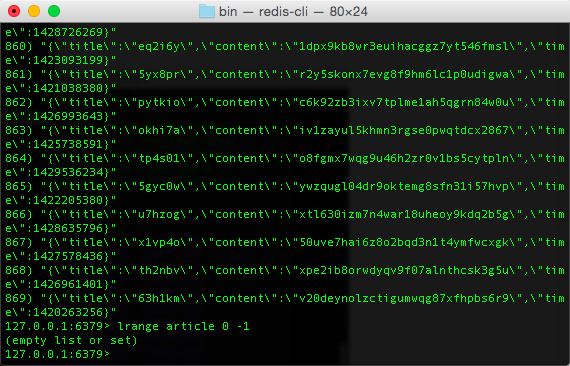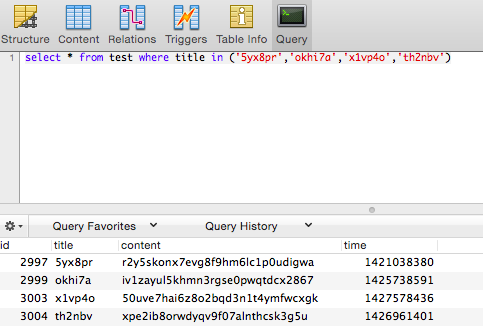# redis实现简单的消息队列(php)

2016/08/15 16:59

$redis = new Redis;$redis->connect('127.0.0.1');
$redis->auth('rainn1234');$article = get_article();
$redis->rpush('article',json_encode($article));

function get_article(){
$shuffle = "abcdefghigklmnopqrstuvwxyz1234567890";$title   = substr(str_shuffle($shuffle), 0,6);$content = substr(str_shuffle($shuffle), 6);$time    = 1420000000 + rand(1,10000000);
return array('title' => $title, 'content' =>$content, 'time' => $time); }$redis->close();

$pdo = new PDO("mysql:host=localhost;dbname=1xin","root","root");$redis = new Redis;
$redis->connect('127.0.0.1');$redis->auth('rainn1234');
while(true){
if ($info =$redis->lpop('article')){
$article = json_decode($info,true);
$sql = "INSERT INTO test(title,content,time) VALUES('{$article['title']}','{$article['content']}',{$article['time']})";
$pdo->exec($sql);
} else {
sleep(1);		//队列中没有消息时，睡眠1s，让出cpu给其他进程
}
}
$pdo->close();$redis->close();1
1 收藏

### 作者的其它热门文章0 评论
1 收藏
1